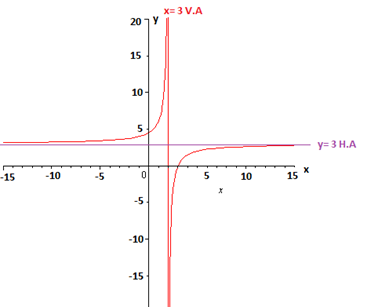# Let f(x) = \frac{3x - 9}{x - 2} (a) state the domain (b) state the vertical asymptote(s) (c)...

## Question:

Let {eq}f(x) = \frac{3x - 9}{x - 2} {/eq}

(a) state the domain

(b) state the vertical asymptote(s)

(c) State the horizontal asymptote

(d) state the graph

## Domain, Asymptotes, and Graph of a Rational Function:

The domain of a rational function consists of all those values except those that annul the denominator. These values are vertical asymptotes and serve us to study the behavior of {eq}f {/eq} and thus graph it.

Let {eq}f(x) = \dfrac{3x - 9}{x - 2} {/eq}

(a) Domain {eq}f= R-\left \{ 2 \right \} {/eq}

(b) Asymptote vertical: {eq}x=2 {/eq}, since this value cancels out the denominator.

Let's study the sign

{eq}\lim\limits_{x \to 2} f(x)= \left\{\begin{matrix} \lim\limits_{x \to 2^+} \dfrac{3x-9}{x-2}= \dfrac{3(2.01)-9}{2.01 -2}= \dfrac{-}{+}= -\infty \\ \lim\limits_{x \to 2^-} \dfrac{3x-9}{x-2}= \dfrac{3(1.99)-9}{1.99 -2}= \dfrac{-}{-}= + \infty \end{matrix}\right.{/eq}

(c) The horizontal asymptote is obtained by making x tend towards infinity. We see this

{eq}\begin{align*} \lim\limits_{x \to \infty } f(x)&= \lim\limits_{x \to \infty } \dfrac{3x-9}{x-2}\\[2ex] &= \lim\limits_{x \to \infty } \dfrac{\dfrac{3x}{x}-\dfrac{9}{x}}{\dfrac{x}{x}-\dfrac{2}{x}}\\[2ex] &= \lim\limits_{x \to \infty } \dfrac{3-\dfrac{9}{x}}{1-\dfrac{2}{x}} \\[2ex] &= 3. \end{align*} {/eq}

So the horizontal asymptote is {eq}y= 3 .{/eq}

(d) Graph of {eq}f {/eq}.